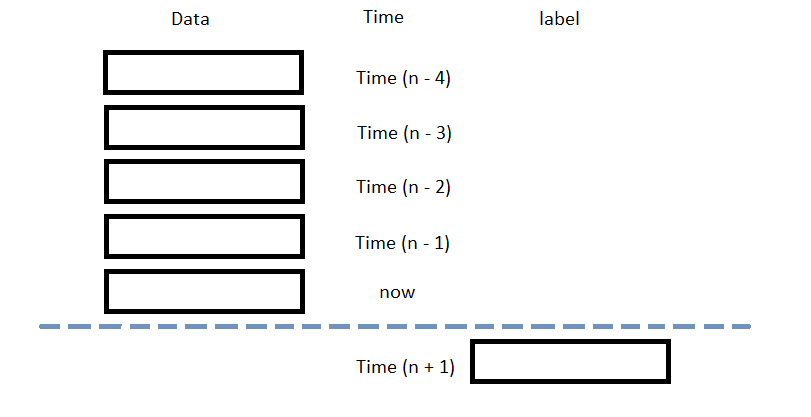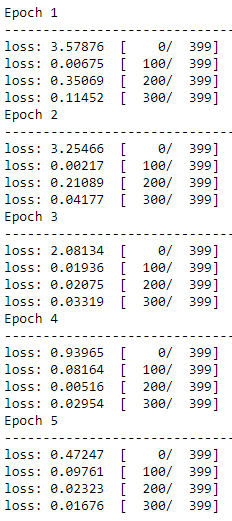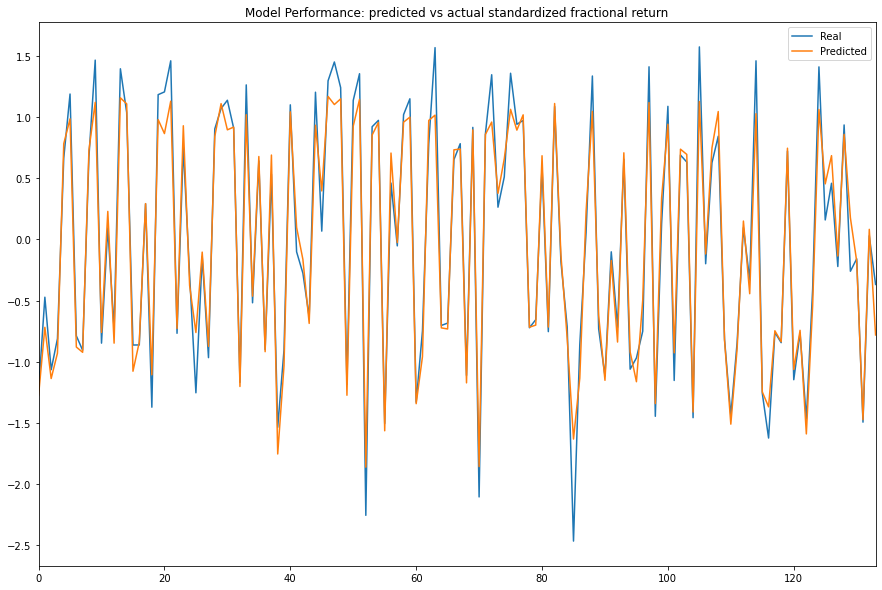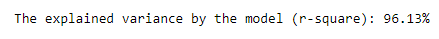# Machine Learning

## PyTorch

### Introduction

This page explains how how to build, train, test, and store PyTorch models.

### Import Libraries

Import the torch, sklearn, and joblib libraries by the following:

import torch
from torch import nn
from sklearn.model_selection import train_test_split
import joblib

You need the sklearn library to prepare the data and the joblib library to store models.

### Get Historical Data

Get some historical market data to train and test the model. For example, to get data for the SPY ETF during 2020 and 2021, run:

qb = QuantBook()
history = qb.History(symbol, datetime(2020, 1, 1), datetime(2022, 1, 1)).loc[symbol]

### Prepare Data

You need some historical data to prepare the data for the model. If you have historical data, manipulate it to train and test the model. In this example, use the following features and labels:

Data CategoryDescription
FeaturesThe last 5 closing prices
LabelsThe following day's closing price

The following image shows the time difference between the features and labels:Follow these steps to prepare the data:

1. Perform fractional differencing on the historical data.
2. df = (history['close'] * 0.5 + history['close'].diff() * 0.5)[1:]

Fractional differencing helps make the data stationary yet retains the variance information.

3. Loop through the df DataFrame and collect the features and labels.
4. n_steps = 5
features = []
labels = []
for i in range(len(df)-n_steps):
features.append(df.iloc[i:i+n_steps].values)
labels.append(df.iloc[i+n_steps])
5. Convert the lists of features and labels into numpy arrays.
6. features = np.array(features)
labels = np.array(labels)
7. Standardize the features and labels
8. X = (features - features.mean()) / features.std()
y = (labels - labels.mean()) / labels.std()
9. Split the data into training and testing periods.
10. X_train, X_test, y_train, y_test = train_test_split(X, y)

### Train Models

You need to prepare the historical data for training before you train the model. If you have prepared the data, build and train the model. In this example, create a deep neural network with 2 hidden layers. Follow these steps to create the model:

1. Define a subclass of nn.Module to be the model.
2. In this example, use the ReLU activation function for each layer.

class NeuralNetwork(nn.Module):
# Model Structure
def __init__(self):
super(NeuralNetwork, self).__init__()
self.flatten = nn.Flatten()
self.linear_relu_stack = nn.Sequential(
nn.Linear(5, 5),   # input size, output size of the layer
nn.ReLU(),         # Relu non-linear transformation
nn.Linear(5, 5),
nn.ReLU(),
nn.Linear(5, 1),   # Output size = 1 for regression
)

# Feed-forward training/prediction
def forward(self, x):
x = torch.from_numpy(x).float()   # Convert to tensor in type float
result = self.linear_relu_stack(x)
return result
3. Create an instance of the model and set its configuration to train on the GPU if it's available.
4. device = 'cuda' if torch.cuda.is_available() else 'cpu'
model = NeuralNetwork().to(device)
5. Set the loss and optimization functions.
6. In this example, use the mean squared error as the loss function and stochastic gradient descent as the optimizer.

loss_fn = nn.MSELoss()
learning_rate = 0.001
optimizer = torch.optim.SGD(model.parameters(), lr=learning_rate)
7. Train the model.
8. In this example, train the model through 5 epochs.

epochs = 5
for t in range(epochs):
print(f"Epoch {t+1}\n-------------------------------")

# Since we're using SGD, we'll be using the size of data as batch number.
for batch, (X, y) in enumerate(zip(X_train, y_train)):
# Compute prediction and loss
pred = model(X)
real = torch.from_numpy(np.array(y).flatten()).float()
loss = loss_fn(pred, real)

# Backpropagation
loss.backward()
optimizer.step()

if batch % 100 == 0:
loss, current = loss.item(), batch
print(f"loss: {loss:.5f}  [{current:5d}/{len(X_train):5d}]")### Test Models

You need to build and train the model before you test its performance. If you have trained the model, test it on the out-of-sample data. Follow these steps to test the model:

1. Predict with the testing data.
2. predict = model(X_test)
y_predict = predict.detach().numpy()   # Convert tensor to numpy ndarray
3. Plot the actual and predicted values of the testing period.
4. df = pd.DataFrame({'Real': y_test.flatten(), 'Predicted': y_predict.flatten()})
df.plot(title='Model Performance: predicted vs actual standardized fractional return', figsize=(15, 10))
plt.show()5. Calculate the R-square value.
6. r2 = 1 - np.sum(np.square(y_test.flatten() - y_predict.flatten())) / np.sum(np.square(y_test.flatten() - y_test.mean()))
print(f"The explained variance by the model (r-square): {r2*100:.2f}%")### Store Models

You can save and load PyTorch models using the Object Store.

#### Save Models

Don't use the torch.save method to save models because the tensor data will be lost and corrupt the save. Follow these steps to save models in the Object Store:

1. Set the key name of the model to be stored in the Object Store.
2. model_key = "model"
3. Call the GetFilePath method with the key.
4. file_name = qb.ObjectStore.GetFilePath(model_key)

This method returns the file path where the model will be stored.

5. Call the dump method with the model and file path.
6. joblib.dump(model, file_name)

If you dump the model using the joblib module before you save the model, you don't need to retrain the model.

You must save a model into the Object Store before you can load it from the Object Store. If you saved a model, follow these steps to load it:

1. Call the ContainsKey method.
2. qb.ObjectStore.ContainsKey(model_key)

This method returns a boolean that represents if the model_key is in the Object Store. If the Object Store does not contain the model_key, save the model using the model_key before you proceed.

3. Call the GetFilePath method with the key.
4. file_name = qb.ObjectStore.GetFilePath(model_key)

This method returns the path where the model is stored.

5. Call the load method with the file path.
6. loaded_model = joblib.load(file_name)

This method returns the saved model.

You can also see our Videos. You can also get in touch with us via Discord.